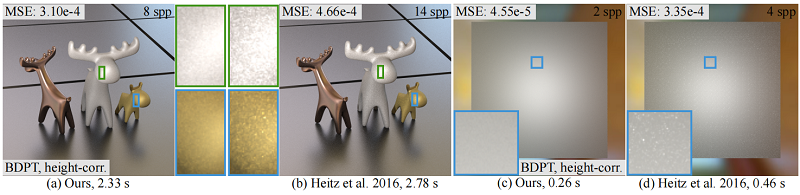# Position-free Multiple-bounce Computations for Smith Microfacet BSDFs

Beibei Wang, Wenhua Jin, Jiahui Fan, Jian Yang, Nicolas Holzschuch, Ling-Qi Yan
ACM Transactions on Graphics (Proceedings of SIGGRAPH 2022)We present a new method to compute multiple bounces for microfacet BSDFs. Our method uses a position-free approach, eliminating the need to trace the heights of previous approaches, significantly reducing noise (see insets). It applies to btoh rough conductors and rough dielectrics. (a) and (b): equal-time comparison with Heitz et al.  for rough conductors (from left to right: copper (GGX model, alpha = 0.1), aluminum (GGX model, alpha = 0.6) and gold (GGX model, alpha = 0.5)). (c) and (d): comparison with Heitz et al.  for a rough dielectric (GGX model, alpha = 1.0). We support both height-correlated and height-uncorrelated shadowing-masking functions. This figure uses the height-correlated model.

# Abstract

Bidirectional Scattering Distribution Functions (BSDFs) encode how a material reflects or transmits the incoming light. The most commonly used model is the microfacet BSDF. It computes the material response from the microgeometry of the surface assuming a single bounce on specular microfacets. The original model ignores multiple bounces on the microgeometry, resulting in an energy loss, especially for rough materials. In this paper, we present a new method to compute the multiple bounces inside the microgeometry, eliminating this energy loss. Our method relies on a position-free formulation of multiple bounces inside the microgeometry. We use an explicit mathematical definition of the path space that describes single and multiple bounces in a uniform way. We then study the behavior of light on the different vertices and segments in the path space, leading to a reciprocal multiple-bounce description of BSDFs. Furthermore, we present practical, unbiased Monte Carlo estimators to compute multiple scattering. Our method is less noisy than existing algorithms for computing multiple scattering. It is almost noise-free with a very-low sampling rate, from 2 to 4 samples per pixel (spp).# BibTex

```@article{Wang:2022:MBBRDF,
author = {Beibei Wang and Wenhua Jin and Jiahui Fan and Jian Yang and Nicolas Holzschuch and Ling-Qi Yan},
title = {Position-free Multiple-bounce Computations for Smith Microfacet
BSDFs},
journal ={ACM Transactions on Graphics (Proceedings of SIGGRAPH 2022)},
year = {2022},
volume = {41},
number = {4},
pages={134:1--134:14}
}
```